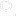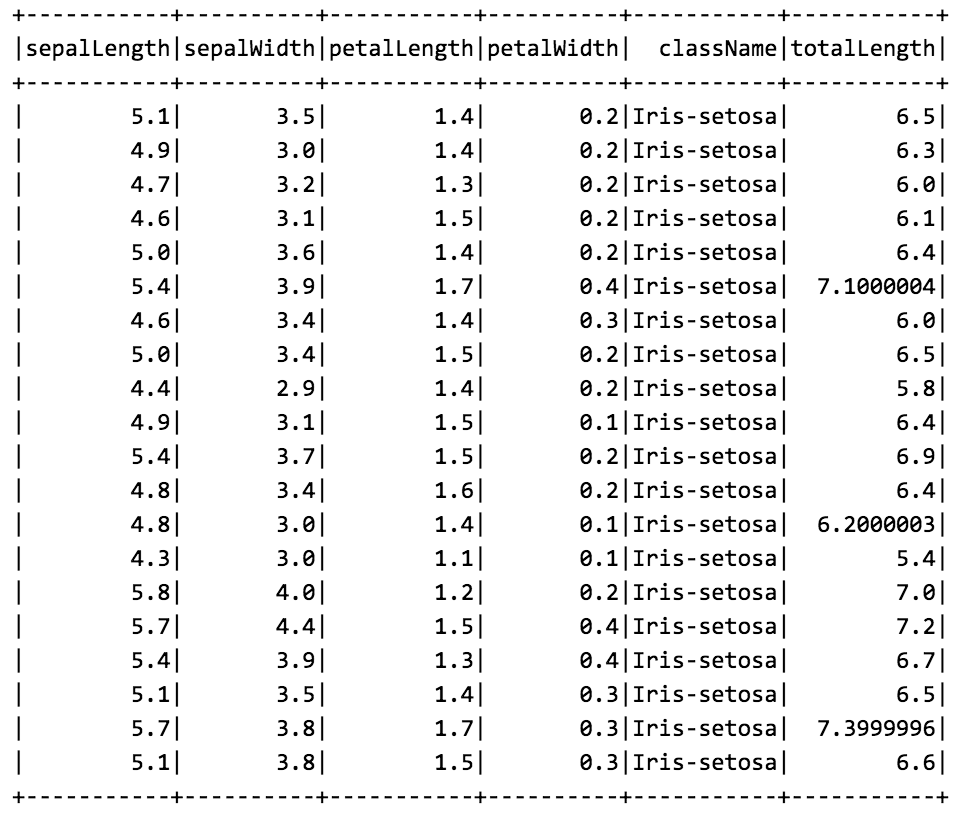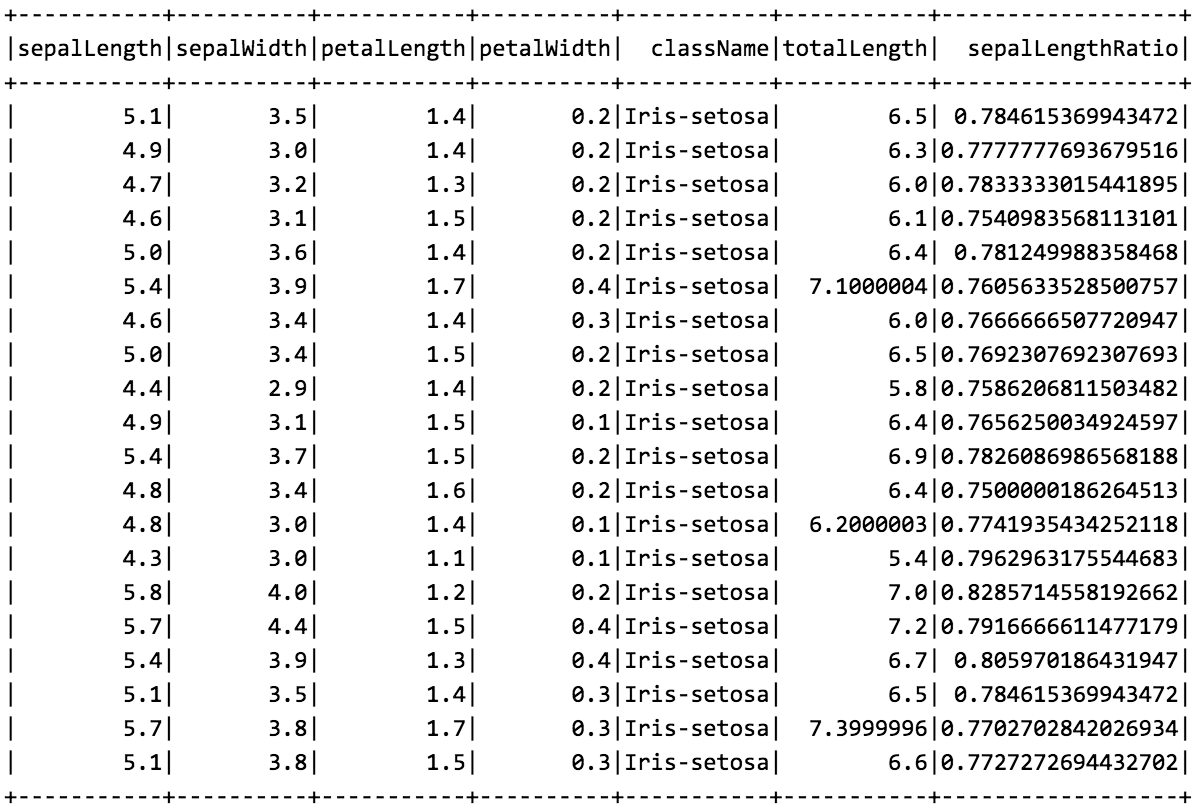# Spark Hot Potato: Passing DataFrames Between Scala Spark and PySpark## Introduction

This blog introduces some of the innovative techniques the CrowdStrike Data Science team is using to address the unique challenges inherent in supporting a solution as robust and comprehensive as the CrowdStrike Falcon® platform. We plan to offer more blogs like this in the future.

CrowdStrike® is at the forefront of Big Data technology, generating over 100 billion events per day, which are then analyzed and aggregated by our various cloud components. In order to process such a large volume of event data, the CrowdStrike Data Science team employs Spark for feature extraction and machine learning model prediction. The results are provided as detection mechanisms for the CrowdStrike Falcon® platform. An early approach is outlined in our Valkyrie paper, where we aggregated event data at the hash level using PySpark and provided malware predictions from our models.

PySpark is an incredibly useful wrapper built around the Spark framework that allows for very quick and easy development of parallelized data processing code. With the advent of DataFrames in Spark 1.6, this type of development has become even easier. However, due to performance considerations with serialization overhead when using PySpark instead of Scala Spark, there are situations in which it is more performant to use Scala code to directly interact with a DataFrame in the JVM. In this blog, we will explore the process by which one can easily leverage Scala code for performing tasks that may otherwise incur too much overhead in PySpark. For this exercise, we are employing the ever-popular iris dataset. We will also use Spark 2.3.0 and Scala 2.11.8.

## Scala Code

First, we must create the Scala code, which we will call from inside our PySpark job. The class has been named PythonHelper.scala and it contains two methods: getInputDF(), which is used to ingest the input data and convert it into a DataFrame, and addColumnScala(), which is used to add a column to an existing DataFrame containing a simple calculation over other columns in the DataFrame. After we have developed our Scala code, we will build and package the jar file for use with the job using: sbt assembly

This will, by default, place our jar in a directory named target/scala_2.11/.

Full Scala source:

package com.crowdstrike.dsci.sparkjobs

import org.apache.spark.SparkContext
import org.apache.spark.sql.{SparkSession, DataFrame}

object PythonHelper {

 // Case class for the data we want to turn into a DataFrame
 case class Data(
 sepalLength: Float,
 sepalWidth: Float,
 petalLength: Float,
 petalWidth: Float,
 className: String
 )

 def getInputDF(sc: SparkContext, infile: String): DataFrame = {
 val spark = SparkSession.builder.getOrCreate()
 import spark.implicits._

 // Get input data
 val inputRDD = sc.textFile(infile)

 // Map each item in the input RDD to a case class using the components
 // of each line
 val outData = inputRDD.map{ line =>

 val Array(sepalLength,
 sepalWidth,
 petalLength,
 petalWidth,
 className) = line.trim().split(',')

 Data(sepalLength.toFloat,
 sepalWidth.toFloat,
 petalLength.toFloat,
 petalWidth.toFloat,
 className)
 }

 // Convert the RDD of Data objects into a DataFrame and return
 outData.toDF()
 }

 def addColumnScala(df: DataFrame): DataFrame = {
 val spark = SparkSession.builder.getOrCreate()
 import spark.implicits._

 // Add another column, with a simple calculation over the existing
 // columns
 df.withColumn("sepalLengthRatio", $"sepalLength"/$"totalLength")
 }
}

## Python Code

Now that we have some Scala methods to call from PySpark, we can write a simple Python job that will call our Scala methods. This job, named pyspark_call_scala_example.py, takes in as its only argument a text file containing the input data, which in our case is iris.data. It first creates a new SparkSession, then assigns a variable for the SparkContext, followed by a variable assignment for the SQLContext, which has been instantiated with the Scala components from the JVM. Using these variables, we can now call into our Scala methods by accessing the JVM through the SparkContext. We call our getInputDF() function, and pass it the JVM version of the SparkContext, along with the input file we specified in the main function arguments. Our Scala code then executes and reads in the data contained in iris.data, and returns a DataFrame. When the DataFrame makes its way back to Python, we wrap it in a Python DataFrame object, and pass in our SQLContext variable with the JVM components. We now have a Python DataFrame which we can manipulate inside our Python code.

Full Python source:

import sys
from pyspark import StorageLevel, SparkFiles
from pyspark.sql import SparkSession, DataFrame, SQLContext
from pyspark.sql.types import *
from pyspark.sql.functions import udf

def total_length(sepal_length, petal_length):
 # Simple function to get some value to populate the additional column.

 return sepal_length + petal_length

# Here we define our UDF and provide an alias for it.
func = udf(lambda sepal_length, petal_length: total_length(sepal_length, petal_length), FloatType())

def add_column(input_df):
 # We use a UDF to perform our simple function over the columns of interest.

 return input_df.withColumn('totalLength', func(input_df['sepalLength'], input_df['petalLength']))

if __name__ == '__main__':
 infile = sys.argv

 # Create the SparkSession
 spark = SparkSession \
 .builder \
 .appName("PySpark using Scala example") \
 .getOrCreate()

 # SparkContext from the SparkSession
 sc = spark._sc

 # SQLContext instantiated with Java components
 sqlContext = spark._wrapped

 # Here we call our Scala function by accessing it from the JVM, and
 # then convert the resulting DataFrame to a Python DataFrame. We need
 # to pass the Scala function the JVM version of the SparkContext, as
 # well as our string parameter, as we're using the SparkContext to read
 # in the input data in our Scala function. In order to create the Python
 # DataFrame, we must provide the JVM version of the SQLContext during the
 #call to DataFrame creation.
 input_df = DataFrame(sc._jvm.com.crowdstrike.dsci.sparkjobs.PythonHelper.getInputDF(sc._jsc.sc(), infile), sqlContext)

 # We now have a Python DataFrame to work with, to which we will add a column.
 transformed_df = add_column(input_df)

 transformed_df.show()

 # We can also perform additional operations on our DataFrame in Scala;
 # here we again access a function from the JVM, and pass in the JVM
 # version of our Python DataFrame through the use of the _jdf property.
 # We receive a DataFrame back from Scala, and then create a Python DataFrame
 # to house it in, again passing in the JVM SQLContext for the DataFrame creation.
 scala_added_df = DataFrame(sc._jvm.com.crowdstrike.dsci.sparkjobs.PythonHelper.addColumnScala(transformed_df._jdf), sqlContext)

 scala_added_df.show()

See how PySpark interacts with the JVM here.

Since we are trying to avoid Python overhead, we want to take a different approach than pulling the entire DataFrame into an RDD, which is shown here:

input_df.rdd.map(lambda row: (row.sepalLength,
 row.sepalWidth,
 row.petalLength,
 row.petalWidth,
 row.className,
 total_length(row.sepalLength, row.petalLength)))

Instead, we will use a UDF to operate on the columns we are interested in and then add a column to the existing DataFrame with the results of this calculation. We define our function total_length(), which performs a simple calculation over two columns in the existing DataFrame. We also define an alias called func, which declares our function as a UDF and that it returns a float value. We are then able to use the withColumn() function on our DataFrame, and pass in our UDF to perform the calculation over the two columns. We can run the job using spark-submit like the following:

spark-submit --jars target/scala_2.11/PySparkScalaExample-0.1.jar pyspark_call_scala_example.py iris.data

Here is the output of transformed_df.show():We now have a Python DataFrame that contains an additional computed column, which we can use for further processing. In order to demonstrate calling Scala functions on an existing Python DataFrame, we will now utilize our second Scala function, addColumnScala() in the same way we accessed the last one, using the SparkContext. This time we will only pass in the JVM representation of our existing DataFrame, which the addColumnScala() function will use to compute another simple calculation and add a column to the DataFrame. We will again wrap the returned JVM DataFrame into a Python DataFrame for any further processing needs and again, run the job using spark-submit:

spark-submit --jars target/scala_2.11/PySparkScalaExample-0.1.jar pyspark_call_scala_example.py iris.data

Here is the output of scala_added_df.show():## Conclusion

We have successfully leveraged Scala methods from PySpark and in doing so have shown mechanisms to improve overall processing time. This strategy is especially useful in cases where one or more processing steps require heavier computation, access to JVM-based libraries, custom types or formats, etc., but which ultimately result in some modification that can be applied to a DataFrame. Since Scala is not a primary language for many Data Science practitioners, this methodology allows for a nice synthesis — with a data engineering team writing libraries that are more performant in Scala and then providing them to a data science team that may be far more familiar with a Python-based environment.

Learn more about CrowdStrike Threat GraphTM, the brains behind the Falcon platform, analyzing over 100 billion event per day.Try CrowdStrike Free for 15 Days